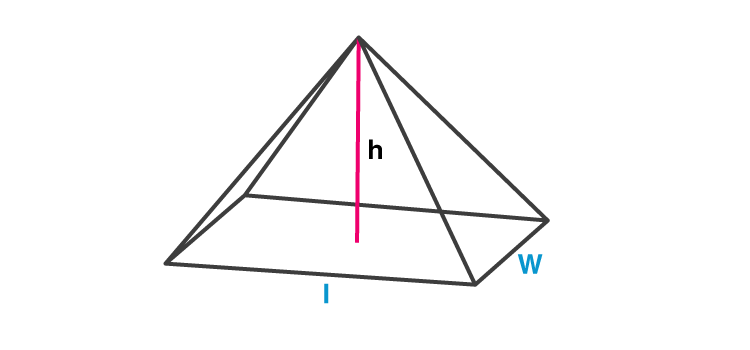# Rectangular Pyramid

Pyramids are three-dimensional structures having triangle faces and have an encompassing polygon shape at its base. If the base of a pyramid is rectangular, then it is called a rectangular pyramid. Though the shape of the base is rectangular, the sides of all pyramids are in a triangular shape. So, whenever we see a pyramid, it resembles a triangle from each of its sides. The shape of the pyramid will help us to determine the surface area and volume of the pyramid.

Like other shapes, we have learned so far in geometry, the pyramid is also defined by their properties. The major properties of the rectangular pyramid are based on edges, faces and vertices.

## Definition

As discussed in the introduction, a rectangular pyramid is a pyramid, which has a rectangular-shaped base. If we look at the bottom view of this pyramid, it looks like a rectangle. Hence, the base has two parallel sides equal.

A pyramid crowns at a point on top of the base, which is known as the apex. A rectangular pyramid can be a right pyramid or oblique pyramid. If it is a right rectangular pyramid, then the apex lies right over the center of the base and if it is an oblique rectangular pyramid, the apex is not directly over the center of the base, but at some angle from the center.Apart from the rectangular pyramid, there are other types of pyramids based on the shape of their bases, such as:

• Triangular Pyramid
• Square Pyramid
• Pentagonal Pyramid
• Hexagonal Pyramid

## Faces, Edges and Vertices

The faces, edges and vertices of any pyramid are their key features. Let us discuss these three properties of the rectangular pyramid.

Faces: A rectangular pyramid has a total of five faces. One face is its rectangular base and the rest four faces are its four triangular-shaped faces. All the triangular faces here are congruent to the opposite triangular face.

Vertex: There is a total of five vertices of a rectangular pyramid. These vertices are the point where the edges meet or intersect. One of the vertices is at the top above the base, where the triangular faces of the pyramid meet. Rest four vertices lie at the corner of the rectangular base.

Edges: A rectangular pyramid has eight edges. Each edge is formed by the intersection of two faces or surfaces. Four out of eight edges are located on the rectangular base, while the other four edges form slopes above the base to a peak point, which is the vertex of the pyramid.

## Rectangular Pyramid Formula

The formula for surface area and volume of a rectangular pyramid is given here:

Surface area of a rectangular pyramid:

 $$A=l w+l \sqrt{\left(\frac{w}{2}\right)^{2}+h^{2}}+w \sqrt{\left(\frac{l}{2}\right)^{2}+h^{2}}$$

Volume of a rectangular pyramid:

 V = (lwh)/3

Where,

l=Lenght of rectangular base

w=width of rectangular base

h=height of pyramid

Test your Knowledge on Rectangular Pyramid